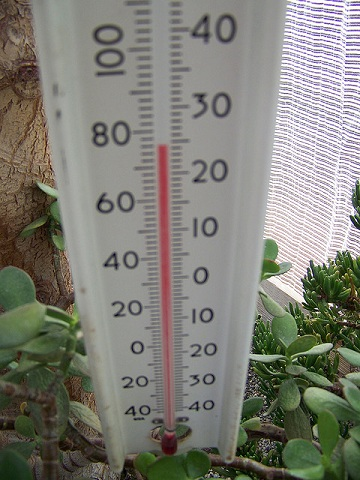# Difference Between Specific Heat and Heat Capacity

## Main Difference – Specific Heat vs. Heat Capacity

Specific heat and heat capacity both describe an amount of energy needed to raise the temperature of a substance. The main difference between specific heat and heat capacity is that specific heat is the amount of energy needed to raise the temperature of a given sample by 1 K while heat capacity is the amount of energy needed to raise the temperature of 1 kg of substance by 1 K.

## What is Heat Capacity

Heat capacity describes the amount of heat needed to raise the temperature of a given sample of a substance by 1 degree Celsius or 1 Kelvin (raising the temperature by 1 oC is the same as raising the temperature by 1 K). Units for measuring specific heat are J oC-1 or J K-1.

## What is Specific Heat

Specific heat ($\mathbf{c}$describes the amount of heat required to raise the temperature of a kilogram of a substance by 1 oC or 1 K. In some fields, the amount of heat required to raise temperature by 1 gram is also often considered.

If a substance having a mass$m$ is given an energy$Q$, and this results in a gain in temperature$\Delta T$ the specific heat is given by:$c=\frac{Q}{m\Delta T}$

The units for specific heat capacity are J kg-1 oC-1 or J kg-1 K-1. The descriptions of heat capacity and specific heat work in the same way for a reduction in temperature by 1 oC or 1 K. Specific heat capacity itself changes as with the temperature. This means that the heat capacity varies, as well. However, for relatively small changes in temperature, the variation of heat capacity can be ignored. At room temperature, for example, water has a specific heat of about 4200 J kg-1 oC-1. This is a comparatively high specific heat, meaning that the amount of energy required to raise the temperature of water by a given amount is also quite high. This fact is extremely useful in regulating temperatures of “warm-blooded” animals.

Another related quantity is molar heat capacity, which describes the amount of energy needed to raise the temperature of 1 mole of a substance by 1 oC or 1 K.## Difference Between Specific Heat and Heat Capacity

### Definition of Specific Heat and Heat Capacity

Heat capacity gives the amount of energy required to raise the temperature of a given sample of a substance by 1 oC.

Specific heat gives the heat capacity per kilogram of a substance.

### Mass Dependence

Heat capacity is directly proportional to the mass of an object.

Specific heat does not depend on the mass of an object, as it is defined per unit mass.

### Units

Heat capacity has SI units J K-1.

Specific heat has SI units J kg-1 K-1.

###### “Thermometer” by jetsandzeppelins (Own work) [CC BY 2.0], via flickr